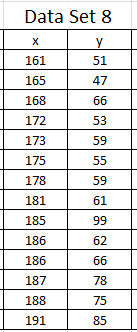# 2464 Scatter Plots Calculating Residuals

78 Questions | Attempts: 239SettingsTesting the assumption of linearity, and calculating residuals.

• 1.
Using Data Set 1, and checking the residual plot, is the assumption of linearity correct in this instance?
• A.

Yes

• B.

No

• 2.
Using Data Set 2, and checking the residual plot, is the assumption of linearity correct in this instance?
• A.

Yes

• B.

No

• 3.
Using Data Set 3, and checking the residual plot, is the assumption of linearity correct in this instance?
• A.

Yes

• B.

No

• 4.
Using Data Set 4, and checking the residual plot, is the assumption of linearity correct in this instance?
• A.

Yes

• B.

No

• 5.
Using Data Set 5, and checking the residual plot, is the assumption of linearity correct in this instance?
• A.

Yes

• B.

No

• 6.
Using Data Set 6, and checking the residual plot, is the assumption of linearity correct in this instance?
• A.

Yes

• B.

No

• 7.
Using Data Set 7, and checking the residual plot, is the assumption of linearity correct in this instance?
• A.

Yes

• B.

No

• 8.
Using Data Set 8, and checking the residual plot, is the assumption of linearity correct in this instance?
• A.

Yes

• B.

No

• 9.
Using Data Set 10, and checking the residual plot, is the assumption of linearity correct in this instance?
• A.

Yes

• B.

No

• 10.
Using Data Set 11, and checking the residual plot, is the assumption of linearity correct in this instance?
• A.

Yes

• B.

No

• 11.
Using Data Set 12, and checking the residual plot, is the assumption of linearity correct in this instance?
• A.

Yes

• B.

No

• 12.
Using Data Set 21, and checking the residual plot, is the assumption of linearity correct in this instance?
• A.

Yes

• B.

No

• 13.
Using Data Set 22, and checking the residual plot, is the assumption of linearity correct in this instance?
• A.

Yes

• B.

No

• 14.
Using Data Set 22, and checking the residual plot, is the assumption of linearity correct in this instance?
• A.

Yes

• B.

No

• 15.
• 16.
The data set above (Data Set 8) shows the height (x) and weight (y) of a team of soccer players. Using the linear regression line, what is the residual to the nearest kg for the player who is 161cm tall?
• 17.
The data set above (Data Set 8) shows the height (x) and weight (y) of a team of soccer players. Using the linear regression line, what is the residual to the nearest kg for the player who is 165cm tall?
• 18.
The data set above (Data Set 8) shows the height (x) and weight (y) of a team of soccer players. Using the linear regression line, what is the residual to the nearest kg for the player who is 168cm tall?
• 19.
The data set above (Data Set 8) shows the height (x) and weight (y) of a team of soccer players. Using the linear regression line, what is the residual to the nearest kg for the player who is 172cm tall?
• 20.
The data set above (Data Set 8) shows the height (x) and weight (y) of a team of soccer players. Using the linear regression line, what is the residual to the nearest kg for the player who is 173cm tall?
• 21.
The data set above (Data Set 8) shows the height (x) and weight (y) of a team of soccer players. Using the linear regression line, what is the residual to the nearest kg for the player who is 175cm tall?
• 22.
The data set above (Data Set 8) shows the height (x) and weight (y) of a team of soccer players. Using the linear regression line, what is the residual to the nearest kg for the player who is 178cm tall?
• 23.
The data set above (Data Set 8) shows the height (x) and weight (y) of a team of soccer players. Using the linear regression line, what is the residual to the nearest kg for the player who is 181cm tall?
• 24.
The data set above (Data Set 8) shows the height (x) and weight (y) of a team of soccer players. Using the linear regression line, what is the residual to the nearest kg for the player who is 186cm tall?
• 25.
The data set above (Data Set 8) shows the height (x) and weight (y) of a team of soccer players. Using the linear regression line, what is the residual to the nearest kg for the player who is 187cm tall?Back to top
×

Wait!
Here's an interesting quiz for you.